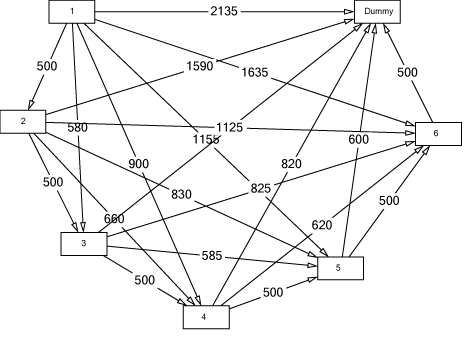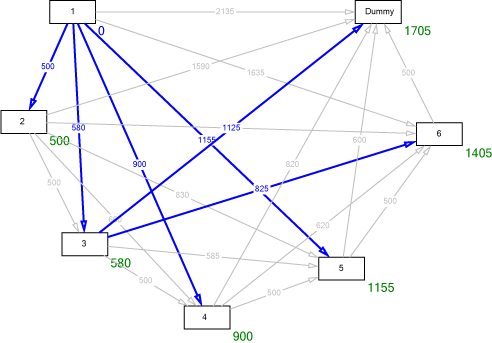### Single-Level Uncapacitated Lotsizing Problem

This problem is also known as the Wagner-Whitin problem.

Assumptions:

 - a single item is considered - demands are dynamic (period-specific, days, weeks, etc.) - the capacity of the resource is neglected

There are several mathematical formulations for this problem. The most common model reads as follows:

$\mathrm{Minimize Z}=\displaystyle{\sum_{t=1}^T \big( {h\cdot y_t}+{ s\cdot \gamma _t} \big)} subject to:$y_{t-1}+q_t-y_t=d_t \qquad {t=1,2,\ldots,T}q_t-M\cdot \gamma _t\le 0 \qquad {t=1,2,\ldots,T}q_t\geq 0 \qquad {t=1,2,\ldots,T}y_t\geq 0 \qquad {t=1,2,\ldots,T}\gamma _t\in \left\{ {0,\,1} \right\} \qquad {t=1,2,\ldots,T}$Symbols: $d_t$demand in period$th$holding costs$M$big number (M must be greater than the maximum lot size)$s$setup costs$T$length of the planning horizon$q_t$lot size in period$ty_t$inventory at the end of period$t\gamma_t$binäry setup variable Every optimum solution to this model satifies the so-called ZIO (zero-inventory-ordering) condition. This means that in any period$t$either the beginning inventory$y_{t-1}$or the lot size$q_t$are zero, i.e.$y_{t-1}\cdot q_t=0$. This property allows to reformulate the above model as a shortest-route model. Example: Consider a product with the following demands:$d_1=20$,$d_2=80$,$d_3=160$,$d_4=85$,$d_5=120$and$d_6=100$. The setup costs are$s=500$and the holding costs are$h=1$. The corresponding shortest-route network is:For each period there is a node. An arrow starting at node$i$and ending at node$j$means: the lot produced in period$i$covers the demand from period$i$to period$j-1$. The numbers associated to the arrows are the setup and holding costs that result from the corresponding lot size. Using any appropriate shortest-route algorithm (e.g. Dijkstra'a algorithm), we get the following solution:Going backwards from node "Dummy" to node "1" we find, that the optimum predecessor of "Dummy" is "3" (production quantity in period 3:$q_3=465$). Next, the optimum predecessor of "3" is "1" (production quantity in period 1:$q_1=100\$). The total costs are 1705.

In industrial practice, heuristic solution procedures are used, such as

• Silver-Meal heuristic
• Least unit-cost heuristic
• Part-period heuristic
• Groff heuristic

and many others.